#Function Repository Resource:

# KeyCombine

Map a function over the keys of an association, and collect or combine values in the event of key collisions

Contributed by: Szabolcs Horvát  |  Szabolcs Horvat
 ResourceFunction["KeyCombine"][f,assoc] maps f over the keys in assoc and collects values having the same key into a list. ResourceFunction["KeyCombine"][f,assoc,comb] uses the combiner function comb to combine values having the same key. ResourceFunction["KeyCombine"][f] represents an operator form of ResourceFunction["KeyCombine"] that can be applied to an expression.

## Details and Options

ResourceFunction["KeyCombine"] works analogously to KeyMap, but it combines values instead of discarding them in the event of key collisions.

## Examples

### Basic Examples (2)

Sort the keys of an association and collect values that end up having the same key:

 In:=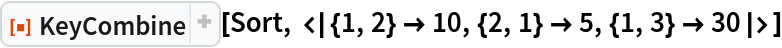Out=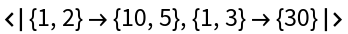Sum values instead of collecting them:

 In:=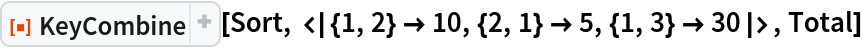Out=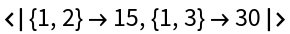### Applications (2)

Convert frequency data (counts) created using a bin size of 1 to a bin size of 3:

 In:=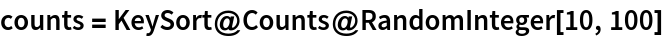Out=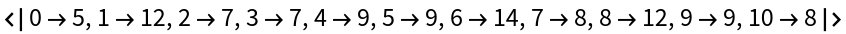In:=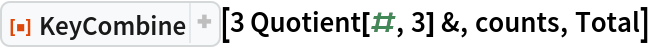Out=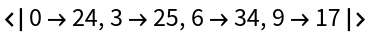Combine reciprocal edges of a weighted, directed graph using geometric mean:

 In:=In:=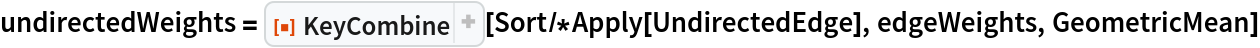Out=In:=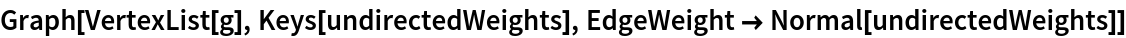Out=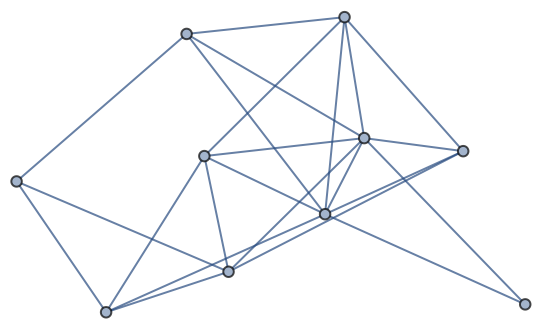### Properties and Relations (1)

KeyCombine does not change the ordering of keys:

 In:=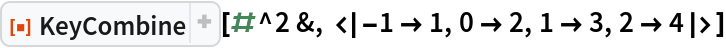Out=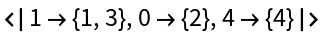## Author Notes

Thank you to Kuba Podkalicki for help with the implementation (see External Links).